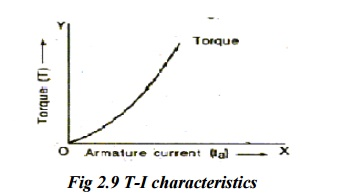Home | | Electrical Drives and Controls | DC Series Motor: Characteristics, Electric Braking

# DC Series Motor: Characteristics, Electric Braking

1. Characteristics of Dc Series Motor 2. Electric Braking in Dc Series Motor

DC SERIES MOTOR

## 1. CHARACTERISTICS OF DC SERIES MOTORS

In dc series motors, the load current drawn from the supply passes through both armature and field windings as they are in series.

o     Therefore when the load on the motor changes, field flux also changes.

o     Hence the characteristics of D.C. series motors entirely differ from the characteristics of D.C. shunt motors.## Torque Vs Armature current characteristics

Ø                 Torque developed in any dc motor T α Ia.

In series motors since field current is equal to armature current. Therefore,

Ø When Ia is small, it is proportional to Ia.

Then torque developed in dc series motor T α Ia2.

Therefore

Ø The torque is proportional to square of the armature current at low values of armature current.

When Ia is large remains constant due to saturation.

Then T a Ia.

Ø Therefore torque is proportional to armature current at large values of armature current. Thus, the torque Vs armature current characteristics begins to rise parabolic ally at low values of armature current and when saturation is reached it becomes a straight line as shown in the following figure.## Speed Vs Armature current characteristics

Consider the speed equation§         When supply voltage V is kept constant, speed of the motor will be inversely proportional to flux.

§         In dc series motors field exciting current is equal to armature current which happened to load current.

§         Therefore at light loads, when saturation is not attained, flux will be proportional to armature current and hence speed will be inversely proportional to the armature current.

§         Hence speed Vs armature current characteristics of dc series motor will be rectangular hyperbola as shown in the following figure.·         As the load on the motor is increased armature current increases and field gets saturated.

·         Once field is saturated flux will be constant irrespective of increase in armature current.

·         Therefore at heavy loads, speed will be constant.

·         This type dc series motor has high starting torque.

## Speed Vs Torque characteristics

The speed Vs Torque characteristics of series motor will be similar to the speed Vs armature current characteristics. It will be a rectangular hyperbola as shown in the following figure.In dc series motors, torque increases with decrease of speed and they are most suitable for operating cranes, lifts, trains, etc.

## D. Rheostatic braking

In this method of breaking, the motor is disconnected from the supply, the field connections are reversed and motor is connected in series with a variable resistance R as shown inThe field connections are reversed to make sure that,the current through the field winding flows in the same direction as before (i.e., from A to B )in order to assist for residual magnetism.

In practice, the variable resistance used for starting purpose is itself used for braking purposes.

The speed-torque characteristics of DC series motor during rheostatic braking is shown in the following figure. explanations are similar to rheostatic braking method applied to DC shunt motor.

## E.  Plugging

In this method of braking, the connections of the armature are reversed and a variable resistance R is put in series with the armature .The above characteristics have been constructed in the same manner as that of plugging conditions applied to DC shunt motor.

## F. Regenerative braking

·         In DC series motor, regenerative braking is not possible without necessary modifications, because reversal of Ia would result in reversal of field and hence of Eb.

·         This method is however used in traction motors with special arrangements.

Study Material, Lecturing Notes, Assignment, Reference, Wiki description explanation, brief detail
Electrical Drives & Control : Drive Motor Characteristics : DC Series Motor: Characteristics, Electric Braking |